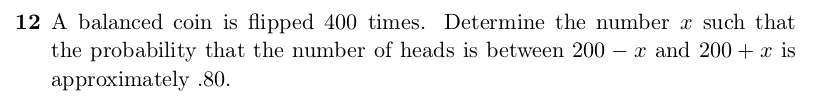We can use the Central Limit Theorem and the Approximating the Binomial probability.

We’re given

• n = 400 trials

• p = 0.5 (and as a fair coin q the probability of failure is also 0.5)

• Probability of number of heads for 400 coin flips between the given intervals is $$\approx 0.8$$

• goal is to find x (or $$S_n^*$$ ) such that $$P((200 - x -0.5 - E[x])/\sigma \leq x \leq (200 + 0.5 + x - E[x])/\sigma) \approx 0.8$$

It is know that the expected value of flipping a coin n times is $$E[x] = np = 400*0.5 = 200$$

The standard deviation $$\sigma = \sqrt{npq} = \sqrt{400*0.5*0.5} = \sqrt{100} = 10$$

As these are 400 trials of a binomial distribution, we standardize the above equation so we can

find the z-score that corresponds to the probability $$\approx 0.8$$ that is \begin{aligned} P(200 - x \leq x \leq 200 + x) \approx P((200 - x - 0.5 - 200)10 \leq x \leq (200 + x + 0.5 - 200)/10) \\ = P((-0.5 - x)/10 \leq x \leq (0.5 + x)/10) \\ \approx NA(-(0.5+x)/10, (0.5+x)/10) \\ \text{For a standard normal distribution as it is symmetric around 0, we can re-write} \\ NA(-(0.5+x)/10, (0.5+x)/10) \\ \text{as} \\ 2*NA(0, (0.5+x)/10)) \approx 0.8 \end{aligned} Dividing 2 on both sides of the equation gives $$NA(0, (0.5+x)/10) \approx 0.4$$

As the area under the normal curve is $$NA(0, (0.5+x)/10) \approx 0.4$$ we can let $$z = (0.5 + x) /10$$ and look up the z value that is $$\approx 0.8$$

and using a standard probability table (there are plenty of websites and books that show it), we find the closest z value

with probability $$\approx 0.8$$ is $$z =1.3$$.

Finally, we can solve for x by solving

$$z = 1.3 =(0.5 + x) / 10$$ which gives $$x = 12.5$$ so this means $$x = 12.5$$ to have the probability that the number of heads be

about 0.8 for 400 fair coin flips.